Martingale Odds in Long Run

by Catalin Barboianu

Anyone knows that odds for the same event to occur several consecutive times in a series of independent plays of a game are very low. This applies for any game of chance, including roulette. For example, hitting the same color for n times in a row on a single-zero roulette has the probability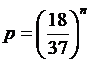. For n = 3, this is 11.51%, for n = 5 is 2.72%, while for n = 10 it goes down to 0.07%.

Basing on these low probabilities of repetition and some mathematical certainties, players built systems and strategies, among which the martingale is the most practiced.

Assuming n consecutive bets are lost and the n + 1 – th is won in a martingale, starting with the amount S as the stake of the first bet, algebra can easily show that the amount received is bigger than the previous cumulated loss, and the profit is S, no matter the value of n.

For a player who runs such system once, the following facts are unquestionable:

- For a sufficient number of consecutive plays, the player always has a positive profit (S);

- For hitting the favorable color and have that profit, the player must sustain the previous cumulated loss (which is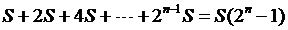).

This cash sustaining is the first issue of the martingale and is a problem of personal money management, because the amounts are not low at all.

As example, for n = 9 (sustaining 9 consecutive failures) and S = \$1 as the first stake, the total amount to loose before the winning bet is \$511. For a \$2 initial stake, the amount to loose becomes \$1022. These are investments for a profit of only \$1 or \$2. Pretty low profit rate, isn't it?

Still, this low profit rate is compensated by the low risk of failure. Only 0.07% is the probability of 10 consecutive color failures, which will ruin a player having only \$511 as capital.

Relying on this low risk, players usually try to extend the use of the martingale over the long run, with the goal of cumulating small positive profits to make an acceptable overall gain.

The error they make stands in their false intuition about having the same risk over the long run as they had in the isolated use of the martingale. Although the color outcomes are independent, when we talk about sequences of consecutive outcomes in a pre-established number of spins, these sequences are not independent any more, so we cannot extend the probability result from an isolated sequence.

Actually, the probability of failure increases significantly over the long run.

Staying with the same example of 9 consecutive failures to sustain, let us evaluate some probabilities of having 10 consecutive failures (the same color for 10 times in a row) over a series of 1000, respectively 10000 spins.

The exact calculation for the probability of having the same color 10 times in a row at least once in 1000 spins is very laborious. We provide here an easier estimation, based on some particular sequences of outcomes. If we split the 1000 spins in 100 consecutive sequences of 10 outcomes (spins 1 – 10, 11 – 20, ..., 91 – 100), we have that these sequences are independent and we have now a Bernoullian probability distribution, which easily allows us to calculate:

- The probability of having exactly one 10-outcome sequence of the same color (choose red, for example) among all 100 sequences is;

- The probability of having exactly two 10-outcome sequences of reds among all 100 sequences is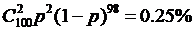;

The next probabilities will become lower, so we can state that the overall probability of having at least one sequence of 10 consecutive reds is higher than 7% (100 times bigger than the initial probability of the isolated case).

In fact, the exact probability of having at least one sequence of 10 reds over 1000 spins (among all 990 sequences, which are not independent) is much higher than 7%.

Making the same Bernoullian calculation for a run of 10000 spins, we get:

- The probability of having exactly one 10-outcome sequence of reds among all 1000  is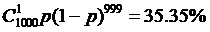;

- The probability of having exactly two 10-outcome sequences of reds among all 1000 sequences is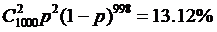;

- The probability of having exactly three 10-outcome sequences of reds among all 1000 sequences is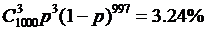and the next probabilities become lower.

Adding them together, we find that the probability of having at least one sequence of 10 consecutive reds is higher than 51% (over 700 times bigger than the initial probability of 0.07%). In fact, the exact probability of having at least one sequence of 10 reds over 10000 spins (among all 9990) is much higher than that.

The conclusion is that the real risk of failure must count in any long-run martingale strategy, since it increases significantly from the isolated case. In addition, the low profit rate of the martingale makes any failure to be a potential ruin. Of course, the player might cumulate enough previous successes to sustain a martingale loss and go ahead, finishing the round with profit. In fact, these conclusions submit to the general consequences of the application of probability theory in gambling: any circumstantial winning is possible in any amount, but toward infinity the player will loose cumulatively in favor of the house. In other words, if we would be immortal regular gamblers, we shall be ruined for sure. Because we are not, there are still chances to get rich or make a living with that. This could be one of the advantages of not being immortal.

Articles  |  Roulette  |  Books